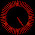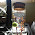## 2016年5月4日 星期三

### 給新手的C++教學 (上冊) - 6. 陣列 (Array)

......

`int array_name[n];`

(心理偷偷的想：耶～小中大括號都用到了耶 \(^o^)/ )

......

「輸入一個整數n，然後輸入n個數字

 ```#include int main() { int n; scanf("%d",&n); int a[n]; int i=0; while(i=0) { printf("%d ",a[i]); i=i-1; } printf("\n"); }``` ```　 ←「i」要從0開始跑，因此先把i設定成0 ←「i」會從0跑到n-1 (這樣會讓程式照順序輸入a～a[n-1]) ←「i」要從n-1開始跑，因此先把i設定成n-1 ←「i」會從n-1往回跑到0 (這樣會讓程式倒序輸出a[n-1]～a) ```

```#include<cstdio>
int main()
{
int a;
int i=1;
while(i<=10)
{
a[i]=0;
i=i+1;
}
int n;
scanf("%d",&n);
i=0;
while(i<n)
{
int v;
scanf("%d",&v);
a[v]=a[v]+1;
i=i+1;
}
i=1;
while(i<=10)
{
printf("%d\n",a[i]);
i=i+1;
}
return 0;
}```

```#include<cstdio>
int main()
{
int n;
scanf("%d",&n);
int a[n];
int i=0;
while(i<n)
{
scanf("%d",&a[i]);
i=i+1;
}
i=0;
while(i<n)
{
int j=i,minimum=i;
while(j<n)
{
if(a[j]<a[minimum])
{
minimum=j;
}
j=j+1;
}
printf("%d ",a[minimum]);
a[minimum]=a[i];
i=i+1;
}
printf("\n");
return 0;
}```

#### 8 則留言:

1.#include <iostream>
using namespace std;
int main()
{
int A={1,2,3,4,5}, B;
for (int i=0;i<10;i++)
A[i]=B[i];

cout<<B;
}

請問一下
我要複製A陣列到B
輸出B=2
但結果為何是0呢？？

1.啊啊我知道了
我寫反了
是B[i]=A[i]

2.哈哈恭喜您自行解決問題 ^_^
加油~~~

3.#include
#include
#include
#include

void shuffle(int wDeck[]);
void deal(const int wDeck[],const char *wFace[], const char *wSuit[]);

//using namespace std;

int main()
{
const char *wFace=
{"Ace","Deuce","Three","Four","Five","Six","Seven",
"Eight","Nine","Ten","Jack","Queen","King"};

int deck={0};
srand(time(0));
int face;
int suit;
shuffle(deck);
deal(deck,face,suit);

}

void shuffle(int wDeck[])
{
int row,column;
for(int card = 1; card <= 52; card++)
{
do
{
row = rand()%4;
column = rand()%13;
}
while(wDeck[row][column] != 0);
wDeck[row][column] = card;
}
}

void deal(const int wDeck[], const char *wFace[], const char *wSuit[])
{
for(int card = 1; card <= 52; card++)

for(int row = 0; row <= 3; row++)

for(int column = 0; column<12 ; column++)

if(wDeck[row][column] == card)
cout<<setw(5)<<setiosflags(ios::right)
<<wFace[column]<<"of"
<<setw(5)<<setiosflags(ios::left)
<<wSuit[row]
<<(card%2 == 0?'\n':'\t');

}
這個請您看一下哪裡錯了?

2.C++的array居然可以動態規劃？！

3.不太了解

int a;
int i=1;
while(i<=10)
{
a[i]=0;
i=i+1;
}

表達的意思

1.int a //a的陣列有11個(0~11)
這整段的意思感覺是初始a~a皆設定為0
可以一個一個設定int a=0,int a=0...
但當有100個的時候,這樣初始比較快!
(以上自我理解~

4.